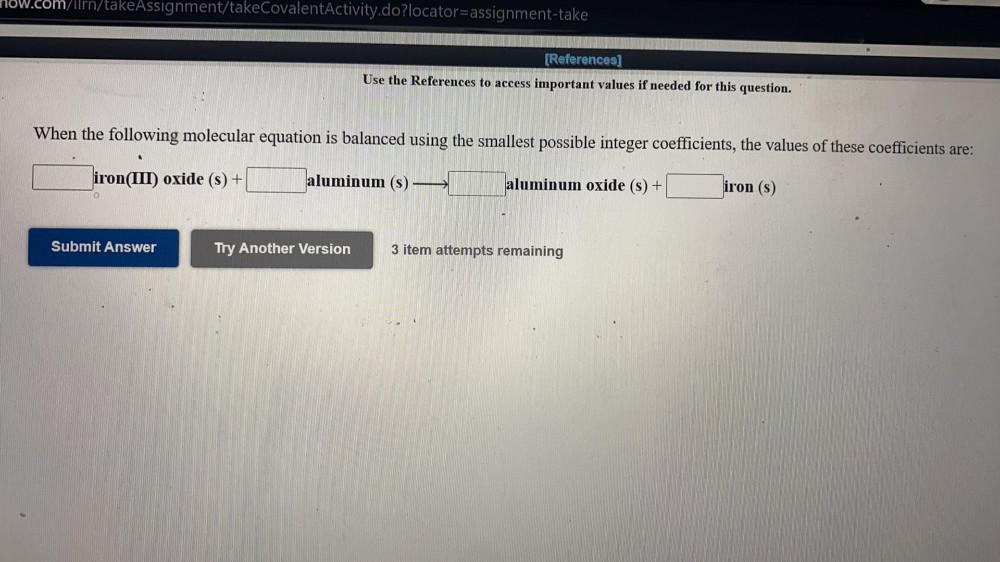Question:

# now.com/ilrn/takeAssignment/takeCovalentActivity.do?locator=assignment-take (References] Use the References to access importantnow.com/ilrn/takeAssignment/takeCovalentActivity.do?locator=assignment-take (References] Use the References to access important values if needed for this question. When the following molecular equation is balanced using the smallest possible integer coefficients, the values of these coefficients are: iron(III) oxide (s) + aluminum (s) aluminum oxide (s) + iron (s) Submit Answer Try Another Version 3 item attempts remaining